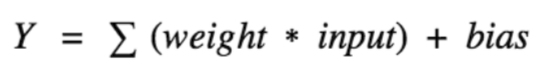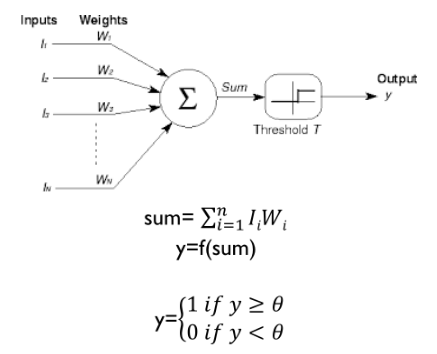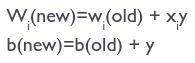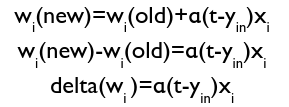# Artificial Neural Network

## Artificial Neural Network

The idea of ANN algorithms is stimulated from the human brain. ANN learn things by processing input information and adjusting weights to forecast the exact output label. We can define a neural network as “It is an interconnected set of neurons, input and output units. Each connection in this interconnected network assigned with weight. These weights are adjusted as per output label in an adaptive manner.”Here, x1, x2 …. xn are input variables, w1,w2….wn are weights for the respective inputs and b is the bias. Y is the output and that is the summation of weighted inputs and bias. After learning and adjusting the weights we apply the activation function to map output to a certain range. The main purpose of the activation function is to introduce non-linearity in the network.

## How neural network works?

An artificial neural network has a set of neurons with input and output units. Multiple neurons were arranged in a layered manner. Each layer is a set of neurons and it implies a stage in the network. Here, each connection is associated with a weight. In the training phase, the ANN learns things by fine-tuning the weights to predict the correct class label of the input tuples.

## Types of Neural Network: Feedforward and Feedback Artificial Neural Networks

Feedforward neural network is a network that is not recursive in nature. Here, neurons in one connected to another layer of neurons but it doesn’t create any cycle, signals travel in only one direction towards the output layer.

Feedback neural networks contain cycles. Here, signals travel in both directions via loops in the network. Feedback neural network is also known as recurrent neural networks.

## Neural Network models

### McCulloch and Pitts Model

In 1943, Warren McCulloch and Walter Pitts created the first mathematical model of an artificial neuron. This simple mathematical model represents a single cell that takes inputs, processes those inputs, and returns an output.### Hebb Network

Hebb network introduced by Donald Hebb in 1949. Hebb network learns by performing the change in the synaptical weight. Here, weights increased in proportion to the product of the input and learning signals.### Perceptron

Perceptron was developed by Frank Rosenblatt in 1950. Perceptron is the simplest supervised neural network that has the capability to learn complex things. Perceptron network consists of three units: Sensory Unit (Input Unit), Associator Unit (Hidden Unit), and Response Unit (Output Unit).

Adaline stands for Adaptive Linear Line. It uses a linear activation function and captures the linear relationship between input and output. For learning, it uses Delta Rule or Least Mean Square(LMS) or Widrow-Hoff rule.### Multi-Layer Perceptron

Multi-Layer Perceptron(MLP) is the simplest type of artificial neural network. It is a combination of multiple perceptron models. Perceptrons are inspired by the human brain and try to simulate its functionality to solve problems. In MLP, these perceptrons are highly interconnected and parallel in nature.

### Backpropagation Network(BPN)

BPN was discovered by Rumelhart, Williams & Honton in 1986. The core concept of BPN is to backpropagate or spread the error from units of output layer to internal hidden layers in order to tune the weights ensures lower error rates.

### Radial Basis Function Networks (RBFN)

RBFN is introduced by M.J.D. Powell in 1985 but Broomhead and Lowe (1988) were the first to exploit the use of radial-basis functions(Gaussian kernel function) in the design of artificial neural networks as a curve-fitting problem. It has a single hidden layer that is non-linear in nature. In RBFN, the function of the hidden layer is different from that of the output layer.

ANN can deal with non-linear and complex problems. It can learn from a variety of problems(such as clustering, classification) and data(such as text, audio, image, videos). ANN has powerful prediction power. It can easily handle the quality issues in the data. It has a high tolerance for noisy data
and has the ability to classify patterns on unseen data.

ANN is a kind of black-box model, lacking in interpretations. It needs a large dataset for training. ANNs always require numerical inputs and non-missing values in the datasets. Tuning neural networks requires a large number of variables.

## Usecases

• Computer Vision: artificial neural networks are capable of solving pattern recognition problems such as object detection, face detection, character recognition, fingerprint recognition, etc.
• Text Mining and Natural Language Processing: Artificial neural networks can be used in various applications in Text Mining and Natural Language Processing tasks such as Text classification, Text Summarization, Named Entity Recognition (NER), Machine Translation, and Spell Correction.
• Anomaly Detection: Artificial neural networks can be used in detecting unusual patterns, outliers, or anomalies in the data.
• Time Series Prediction: Artificial neural networks also useful in dealing with time-series data such as sales forecast, stock price, and weather forecast.

## Summary

Artificial neural networks are complex systems that simulate human brain functionality. It has the capability to solve a variety of data mining problems such as pattern recognition. It offers a black box solution with higher accuracy and less interpretability.